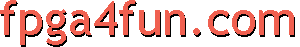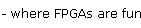EPP 4 - The HDL

Let's see how easy it is to implement an EPP port in an FPGA.

First let's create a few signals from the parallel port signals. We invert some signals to make all new signals active high.

// Let's create a few signals from the "PP" parallel port pins
// first for the EPP outputs (i.e. outputs from the PC, inputs for us)
wire EPP_write = ~PP;
wire EPP_data_strobe = ~PP;
wire [7:0] EPP_datain = PP[9:2];

// now for the EPP inputs
wire EPP_wait;			assign PP = EPP_wait;
wire [7:0] EPP_dataout;		assign PP[9:2] = EPP_dataout;

Now most FPGA designs use their own clock. Let's call our clock "clk" and synchronize the strobe signals to our clock.

// Use a 3-taps shift register to synchronize EPP_strobe to our clock
wire EPP_strobe = EPP_data_strobe | EPP_addr_strobe;  // only one is active at a time
reg [2:0] EPP_strobe_reg;
always @(posedge clk) EPP_strobe_reg <= {EPP_strobe_reg[1:0], EPP_strobe};

// detect the strobe edges
wire EPP_strobe_edge1 = (EPP_strobe_reg[2:1]==2'b01);
wire EPP_strobe_edge2 = (EPP_strobe_reg[2:1]==2'b10);

// respond right away to a transaction
assign EPP_wait = EPP_strobe_reg;

Now we can handle the EPP writes. Let's store the received values into registers.

// EPP writes
always @(posedge clk) if(EPP_strobe_edge1 & EPP_write & EPP_data_strobe) data_reg <= EPP_datain;

EPP reads now. Let's just read back what are stored in the registers...